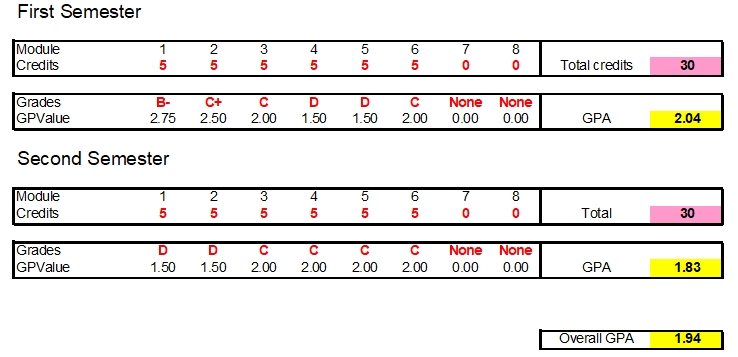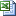## GPA CalculatorDo you want to know how to calculate your GPA? When you are studying at the school or college, it is important to know the average of grade points you have. You will find out your academic progress. It is true that the calculation will be done by the authorized academic department in the college where you are studying at. But it is also necessary for you to make the personal record of the GPA calculation. Do you want to know how?

You can use this GPA Calculator for Excel. It is true that finding this kind of calculating tool on the internet is quite easy. But it is also possible if you want to create your own calculator using Microsoft Excel. You can make 5 columns for number, taken classes, credits, grade and grade points. To fill up the grade points, you have to know the standard grade levels used in your college. The highest grade, A is commonly used to represent 5 or 4. The lowest one will be E or F to represent 0.

Do you want to know how to calculate the GPA? Fill up the columns of number, class, credits and grade. And to know the amount of grade points of each class, you can use IF formula by considering the grade and taken credits. After all columns are filled, sum the credits and grade points. To get to know the GPA, you will just need to divide the total grade points with the sum of the taken credits.

CHECK THIS TEMPLATE >  Home Affordability Calculator

This GPA calculator is free for editing. If you are looking at its formula, you can see that it is built on simple formulas. You can add or duplicate it for other purposes as well.GPA Calculator Template (19.5 KiB, 689 hits)